Mr_Stewart_Maths

•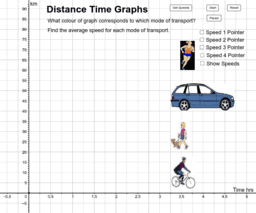Distance Time Graphs

Activity

Mr_Stewart_Maths

•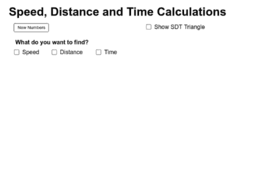Speed Distance Time Calculations

Activity

Mr_Stewart_Maths

•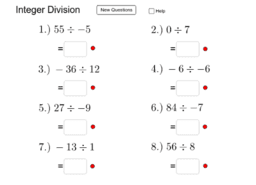Integer Division

Activity

Mr_Stewart_Maths

•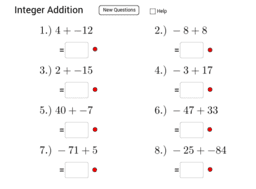Activity

Mr_Stewart_Maths

•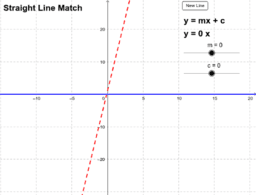Straight Line Match

Activity

Mr_Stewart_Maths

•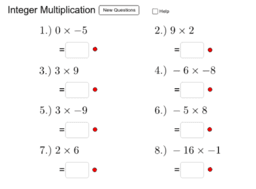Integer Multiplication

Activity

Mr_Stewart_Maths

•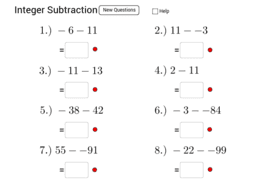Integer Subtraction

Activity

Mr_Stewart_Maths

•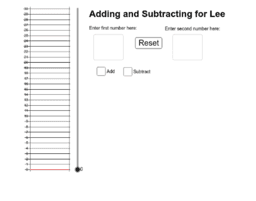add and subtract help for lee

Activity

Mr_Stewart_Maths

•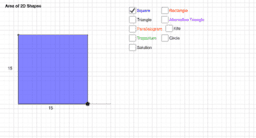Area of 2d Shapes

Activity

Mr_Stewart_Maths

•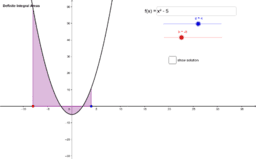Integral Calculus

Book

Mr_Stewart_Maths

•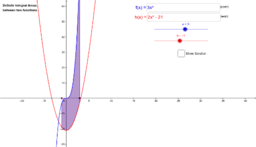Integral Area between functions

Activity

Mr_Stewart_Maths

•Integral Area from graph

Activity

Mr_Stewart_Maths

•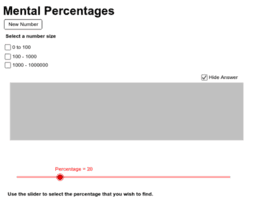Mental Percentages

Activity

Mr_Stewart_Maths

•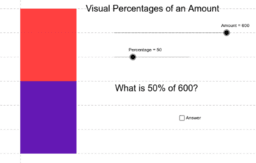Visual Percentages

Activity

Mr_Stewart_Maths

•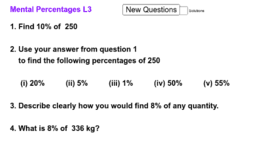Mental Percentages of a quantity L3

Activity

Mr_Stewart_Maths

•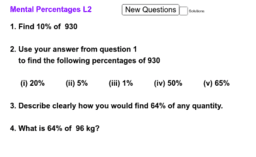Mental Percentages of a quantity L2

Activity

Mr_Stewart_Maths

•Mental Percentages of a quantity

Activity

Mr_Stewart_Maths

•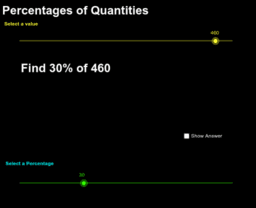Percentages of a quantity

Activity

Mr_Stewart_Maths

•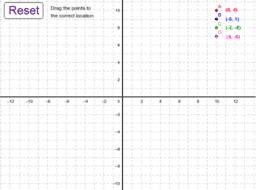Coordinates Symmetry Transformations

Book

Mr_Stewart_Maths

•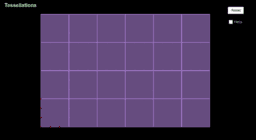Tessellations

Activity

Mr_Stewart_Maths

•Coordinate Game

Activity

Mr_Stewart_Maths

•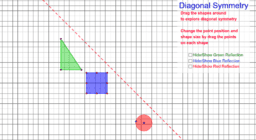Diagonal Symmetry

Activity

Mr_Stewart_Maths

•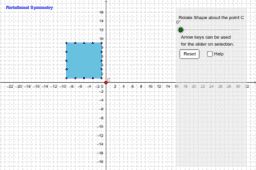Rotational Symmetry

Activity

Mr_Stewart_Maths

•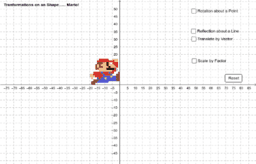Tranformations

Activity

Mr_Stewart_Maths